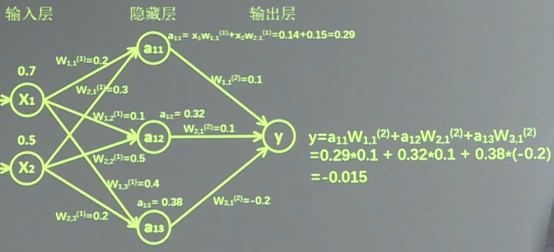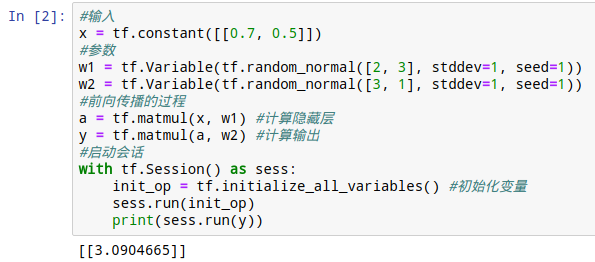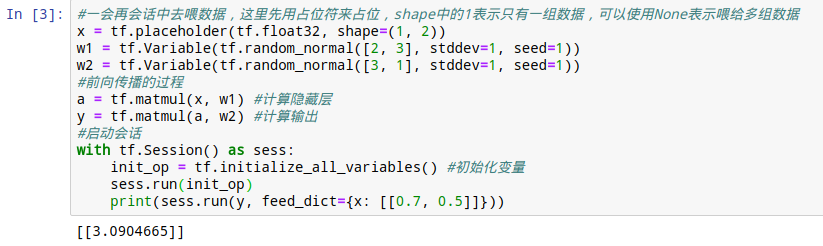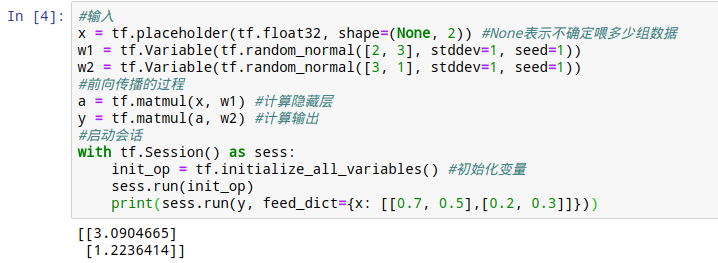# TensorFlow学习笔记（二）--神经网络的前向传播``````#瞎编两个输入特征：0.7和0.5
x = tf.constant([[0.7, 0.5]])
#接下来搭建的是含有一个3神经元的隐藏层的神经网络，所以瞎搞两组权重
#生成正态分布随机数，2*3的矩阵，因为输入特征是两个，隐藏层神经元是3个
w1 = tf.Variable(tf.random_normal([2, 3], stddev=1, seed=1))
#生成3*1的正态分布随机数，因为隐藏层神经元作为特征是3个，输出只有一个
w2 = tf.Variable(tf.random_normal([3, 1], stddev=1, seed=1))``````

``````#前向传播的过程
a = tf.matmul(x, w1) #计算隐藏层
y = tf.matmul(a, w2) #计算输出``````

``````#启动会话
with tf.Session() as sess:
init_op = tf.initialize_all_variables() #在使用变量前必须初始化变量
sess.run(init_op)
print(sess.run(y))````````x = tf.placeholder(tf.float32, shape=(1, 2))``但是这个程序还有个弊病，占位符的shape=(1, 2)中的1如果不知道，即我们不知道输入多少组数据怎么办。只要使用None替换掉1就好了：